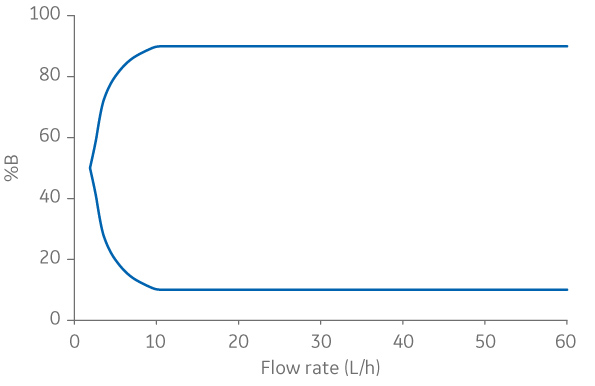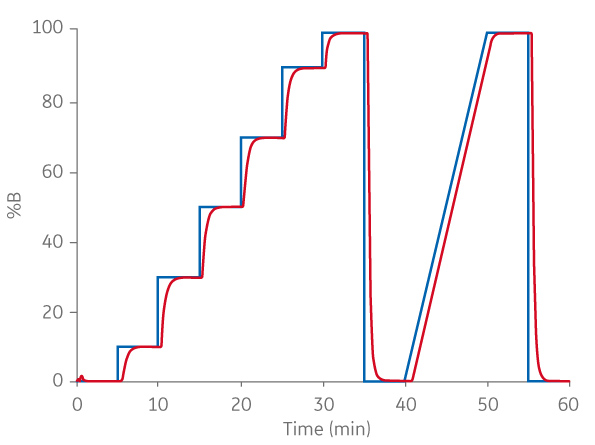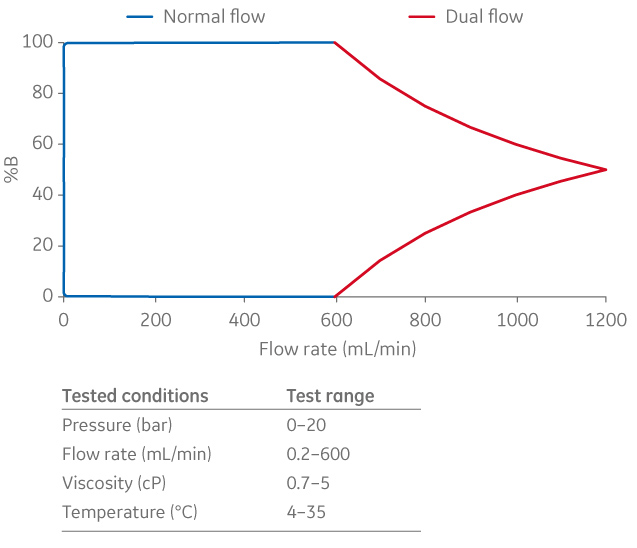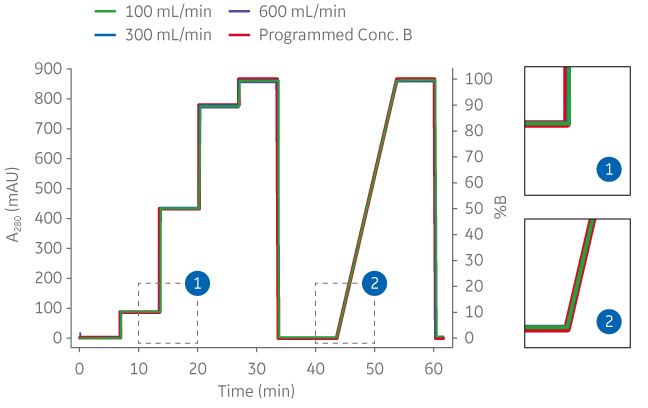Accurate flow and gradient performance are important to provide reproducible chromatography results at different scales. In this study we investigated flow and gradient accuracy of the ÄKTA pilot 600 chromatography system.

## Introduction

The ability to scale up your bioprocess in an efficient way is important to increase speed to market. A comparable flow accuracy between scales can facilitate scaling of your methods. When scaling, it is important that the results (as visualized in the chromatogram) are comparable and reproducible between scales.

Examples of applications where maintained flow accuracy and gradient performance are especially important when scaling up your process are separation of mAb charge variants, of closely related entities, and of proteins of similar charge and size.

For the ÄKTA pilot 600 chromatography system, flow and gradient formation is controlled by the UNICORN system control software. In this work, flow accuracy was investigated at a combination of different backpressures, flow rates, temperatures, and liquid viscosities.

Gradient accuracy and range were calculated from the flow accuracy. Gradient accuracy was also evaluated by running step and linear gradients at different flow rates. Within the buffer B gradient range (%B) from 1%B to 99%B at a system flow rate of 5–600 mL/min, gradient accuracy was found to be ± 1%B.

### ÄKTA pilot 600 chromatography system

ÄKTA pilot 600 is an automated bench-top chromatography system equipped with two high-performance, low-pulsation piston pump modules to ensure accurate and reproducible results (Fig 1). The piston pump modules enable creation of binary gradients with efficient mixing at all flow rates and pressures allowed by the system.

For high pump accuracy, even at low flow rates, and to prevent siphoning when the pumps are not running, a restrictor module is situated directly after the pump modules.

The maximum flow rate of the system is 1200 mL/min when operated in isocratic mode. In gradient mode, the maximum flow rate is 600 mL/min. Maximum operating pressure is 20 bar. This work demonstrates flow accuracy and gradient performance of ÄKTA pilot 600.Fig 1. ÄKTA pilot 600 high-accuracy chromatography system designed to facilitate sanitization (1). 1= pumps, 2 = restrictor, and 3 = column valve.

### Designation of flow accuracy

Flow accuracy can be specified either as percentage of full scale (% FS) or as percentage of reading (% RD), or a combination of both. If an instrument has a flow accuracy specified as % FS, the error will be of a fixed value irrespective of flow rate.

For example, if the full-scale flow rate is 1000 mL/min and the system has an accuracy of 2% FS, the deviation will be ± 20 mL/min at all flow rates. This means that at a flow rate of 100 mL/min, the deviation will also be ± 20 mL/min (or ± 20% deviation from the read value).

If a system instead has a flow accuracy specified as % RD, the error will always be the same percentage of the read value. In such a case, with a system accuracy of 2% RD, the deviation would be ± 20 mL/min at a flow rate of 1000 mL/min, but only ± 2 mL/min at 100 mL/min.

Gradient accuracy is often presented as a gradient acceptance range, with a defined accuracy within the acceptance range or as a chromatogram over a programmed and a performed gradient (Fig 2). The gradient acceptance range is given as % of buffer B (%B) for the flow rate range of the system, and the gradient accuracy is defined as the deviation from %B.

The chromatogram is usually presented as the programmed %B and the performed %B at a single flow rate. The higher the flow rate; the lower the system dead volume; and the longer the gradient formation time, the better the gradient performance of the system.Flow rate (L/h)Fig 2. (A) Example of gradient acceptance range for diaphragm pumps. (B) Example of how diaphragm pump gradient accuracy can be illustrated.

## Results

Flow accuracy of ÄKTA pilot 600 was found to be ± 2% RD. Gradient deviation, within the acceptance range 1%B–99%B, was less than 1%B, at system flow rates of 5–600 mL/min. At dual pump flow rates between 600–1200 mL/min, the gradient accuracy was the same, but the gradient range was limited by the maximum flow rate of 600 mL/min of each pump.

Figure 3 depicts the gradient range at flow rates between 0–1200 mL/min. The gradient formation at low, intermediate, and high flow rates is illustrated in Figure 4.Fig 3. Gradient range of ÄKTA pilot 600. Blue = normal flow, red = dual flow.Fig 4. Chromatograms from ÄKTA pilot 600, showing the programmed and the resulting step and linear gradients at 100, 300, and 600 mL/min, respectively.

## Conclusion

The high accuracy over a multitude of parameters such as flow rate, viscosity, and pressure indicates that this chromatography system has a highly stable performance, omitting the need for a separate flowmeter to feedback control the pumps.

The high accuracy, together with small hold-up volumes, strong sanitary performance (1), and a similar gradient performance as the ÄKTA avant and ÄKTA pure chromatography systems, makes ÄKTA pilot 600 well-suited for scaling, while minimizing the uncertainties associated with process transfer between GMP and non-GMP environments.